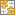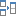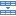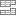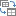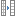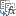# Look up with Lookups in Excel

Helen Bradley explains how to use the vlookup function in Microsoft Excel to find data in a table.

When you need to find information in a table of data the lookup functions in Excel can help you do it. While there was a lookup wizard in earlier versions of Microsoft Excel that made the process of creating a lookup quite easy, this is no longer available in Excel 2010. Now, when you need a lookup formula you'll have to create it manually. In this post, I'll show you how to harness the power of lookups for your Excel worksheets.

### The basics

There are multiple lookup functions in Microsoft Excel and these include vlookup, the very similar hlookup, and lookup. You use vlookup to find data in a table by searching for a value in the first column of the table and then returning the corresponding value in another column.

Use hlookup when the data is organized differently to find a matching value in the top row of a table and to return the corresponding value from one of the rows below. The lookup function has two forms - a vector and an array form and it returns a value from a one column or one row range, or from an array. Of the three, the one you're most likely to use day to day is vlookup and this is the one I'll focus on here. Basically if you can understand and create a vlookup you can create an hlookup function too.

### Vlookup syntax

To use vlookup to return a value from a table you will provide Excel with the value to look for in the first column of the table, the table range and the column which contains the value to return.

When you provide the table range, Excel looks for the value you specify in the first column of that range - typically the first column will be the row headings for your data. When describing the column you do so as the number of the column in the table range you've provided. Column 1 is the first column and 2 is the one immediately to its right and so on. If you specify a column number that is out of the range of your table - such as less than 1 or greater than the number of columns in your table range you'll get an error.

There is one optional True/False argument for this function which lets you force an exact match or an approximate one - the default is the latter. If you specify an exact match (False) then the table need not be sorted. If you do not include this argument or if you specify an approximate match (True) you must sort the table so that the values in the first column are in ascending order - if not - the function may not return the correct result. Where you omit this argument or use True, if there is not an exact match, then Excel uses the nearest value, in the first column, to the value that you are searching for that is smaller than that value.

So, for example, in this table where we are showing pounds weight, a handling and a shipping charge, we can use vlookup to search for a weight and determine the handling and shipping charge for a parcel of that weight. Of course most parcels won't weigh exact amounts so we will use the True value (or omit it) so the formula will return a result even if an exact match is not found. This also means we need to sort the table so the data in the first column is in ascending order.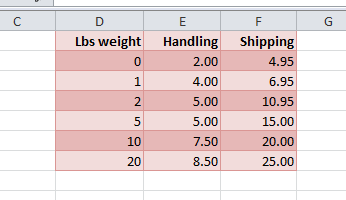### Vlookup at work

If we try to find a match for a 1.5 pounds weight parcel there is no entry for 1.5 pounds. In this case, the lookup function will return the entry for the largest value that is less than the value that we're looking up. So if we're looking up 1.5 and there is no match for it, Excel will look for the number that is nearest 1.51 but less than it - in other words 1.

To look up a value in cell B11 that is the pounds weight of a parcel (in this case 1.5lbs) and to return the handling value, you would write this formula:

=VLOOKUP(B11,D2:F7,2)

The formula returns the handling cost of \$4 - the value in column 2 of the table opposite the next nearest but smaller value to the one we are looking up.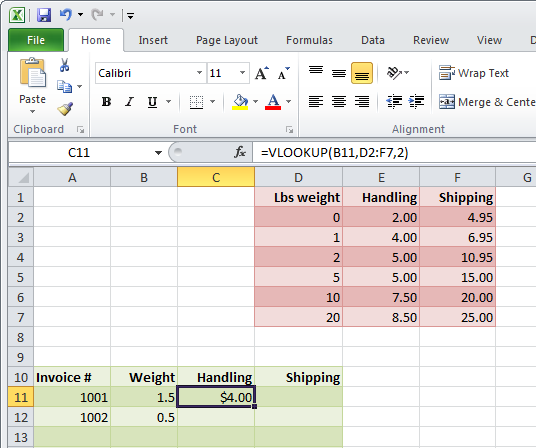If you want to be able to copy the formula down a column, then you will need to alter it so that the table reference is absolute, so it would look like this:

=VLOOKUP(B11,\$D\$2:\$F\$7,2)

Of course, you can also name your table by selecting the cells from D1 to F7 and choose Formulas > Define Name, type a name for the table and click Ok.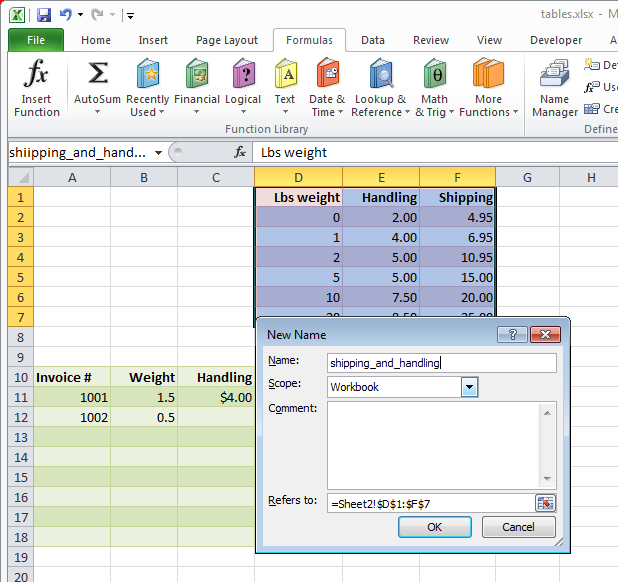Now you can use the table name when you are creating the formula. Here the named range appears in place of the range itself:

=VLOOKUP(B12,shipping_and_handling,2)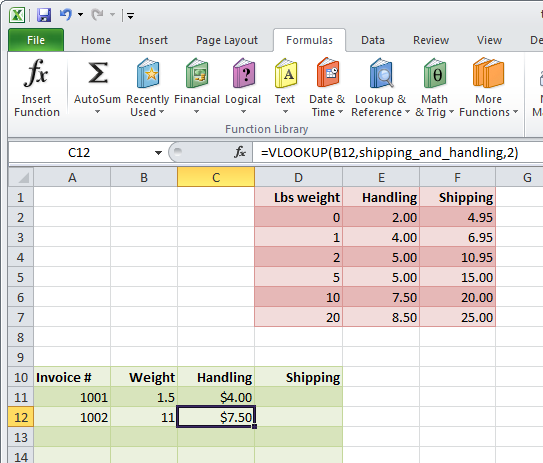We can adapt the formula for the handling to deal with the shipping. In this situation because the shipping is in column 3 of the table, the only change will be to the last value in the formula:

=VLOOKUP(B12,shipping_and_handling,3)

Hlookup works similarly, it takes the same values, in other words the value to look up, the range of the table, but in this case you'll be providing a row number rather than a column number. The rows will be numbered 1, 2, 3 and so on with row 1 being the first row in the table.

In the example above, we want to be able to find weights such as 11, 12 and 25 in a table that simply doesn't have those values in it. The ability to find the closest value that is smaller than the value that we're looking up is extremely attractive. There are, however, some provisos when you're using this process and that is that you'll have to have a starter value as we do of 0. This will catch the situation where you have a shipping weight of less than 1 pound.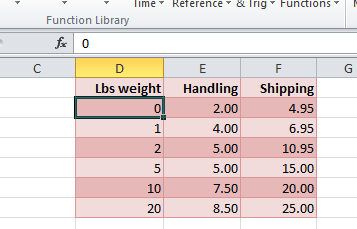### Working with exact matches

In other circumstances you may want an exact match and there may be no room for the next nearest values. The example below for rug sizes and costs is exactly that. If you don't have an order for a green weave rug then there is no next lowest value. In this situation, we want an exact match or an error message.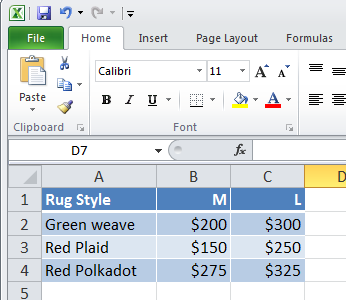For this, we'll look up the value in column A and return a value from column 2 or 3 depending on whether the order is for a medium or a large rug. In this circumstance we'll need to use an If function to determine which column number to use. The lookup function will read as follows:

=VLOOKUP(A7,A2:C4,IF(B7="M",2,3),FALSE)

In this case, we're looking up the value in column A and looking for a match in column B or C depending on whether it's a medium or a large rug. If there is not an exact match, in other words if the rug description being ordered is not a direct match for one of the rug descriptions in column A then a #N/A error will be returned. If the rug size isn't one of the two options it will default to the large size because of the way the IF function is written.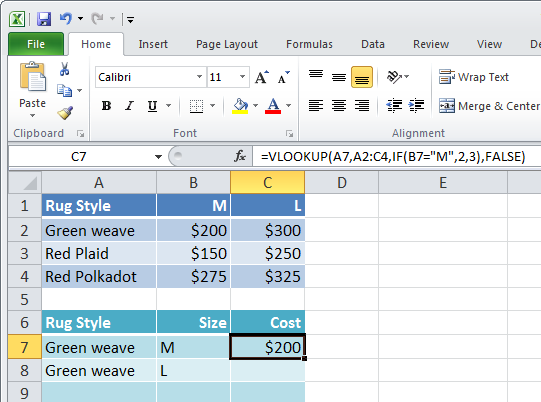### Using data validation

In practical circumstances you may want to be assured that your user will enter one of the listed rug descriptions and sizes. You could do this using a Data Validation List. To do this, select the cells in which the user can place their answer such as column A or B, and choose Data > Data Validation > Data Validation and from the Settings option choose List. In the Source area, click and then drag over cells A2 to A4 which are the list of rug descriptions. Click Ok.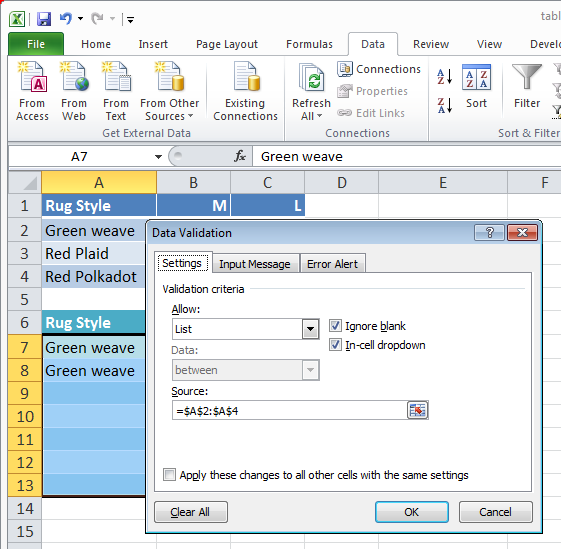You can also create data validation lists to enter the value M or L as the rug size using the data from cells B1:C1 as the source data for the list.

Now when the user goes to enter a rug style they can select an item from the dropdown list. This ensures that they will always spell the name correctly so that they will always choose an item that is in the list. It also means that if they change their selection and choose a different rug, the vlookup function will recalculate automatically and produce the desired cost.

### Sort the data

If you are working with inexact matches you must sort your table. To do this, select all the data in the table including the row headings in the first column. You can select the column headings or not. From the Data tab, select Sort to display the Sort dialog. From the Sort By options choose the first column, choose to sort on Values and choose the sort order which must be A to Z (ascending). If your table has column headers and if you selected these when you selected your data, then check the My Data has Headers checkbox. Click Ok and the data table will be sorted in order so that the vlookup function works correctly.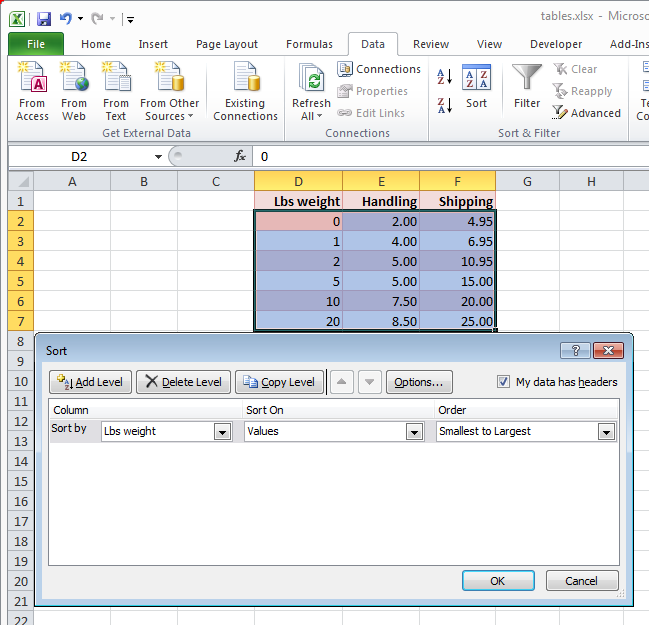### 8 responses to "Look up with Lookups in Excel"

1.mubeen says:

how use to vlookup function at lookup reverse method

2.Atul Jain says:

Thank you for this post.

If I want to get the value of 14 through interpolation

10 : 7.5
20 : 8.5

14 : through interpolation method (in between 7.5 to 8.5)

then how can i get the value ?

3.Atul Jain says:

If I want the value of 11 : 8.5

4.najeeb says:

How to consolidate 30 Excel FIle(Date wise name) in a Excel.

Kindly help

•Svetlana Cheusheva (Ablebits.com Team) says:

Hi Najeeb,

Check out this Consolidation add-in for Excel. It provides a large variety of options to consolidate data from multiple worksheets into one.

•Ibby says:

Thanks Svetlana,
I was looking for this. :)

5.constantin says:

I have firste information:
411 A20 10
411 A21 20
411 A23 10
4220 A20 10
4220 A21 20
550 A10 15
550 A11 33

and I want to bring on the same row information like this:
Account code Olympic Jurnal Magnitude % From jurnal
411 100 40 140 29% A20; A21; A23
4220 40 30 70 43% A20; A21;
550 50 48 98 49% A10; A11;

•Irina Pozniakova (Ablebits.com Team) says:

Hello Constantin,

Our Combine Rows Wizard add-in can bring all data referring to the same Account code to the same row:
https://www.ablebits.com/excel-combine-rows/index.php

However, you show some additional information in the expected results. Could you please specify where you have the data "Olympi Jurnal Magnitude % From jurnal"?